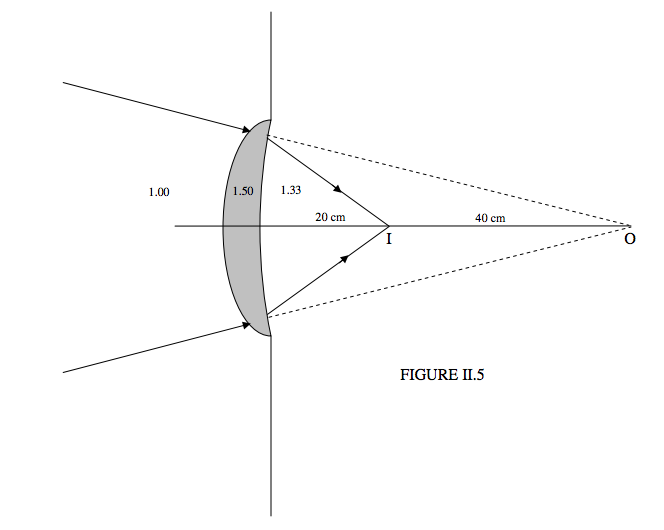$$\require{cancel}$$

# 2.4: Convergence

•• Contributed by Jeremy Tatum
• Emeritus Professor (Physics & Astronomy) at University of Victoria

Figure II.5 shows a lens made of glass of refractive index 1.50. To the left of the lens is air (refractive index 1.00). To the right of the lens is water (refractive index 1.33). A converging beam of light is incident upon the lens directed toward a virtual object O that is 60 cm from the lens. After refraction through the lens, the light converges to a real image I that is 20 cm from the lens.I am not at this stage going to ask you to calculate the radii of curvature of the lens. (You can’t – you need one more item of information.) I just want to use this diagram to define what I mean by convergence.

The convergence of the light at the moment when it is incident upon the lens is called the initial convergence $$C_1$$, and it is defined as follows:

$initial\space convergence = \frac{Refractive\space index}{Object \space distance}. \label{eq:2.4.1}$

The convergence of the light at the moment when it leaves the lens is called the final convergence $$C_2$$, and it is defined as follows:

$final\space convergence = \frac{Refractive\space index}{Image \space distance}. \label{eq:2.4.2}$

Sign convention

• Converging light has positive convergence;
• Diverging light has negative convergence.

Example $$\PageIndex{1}$$

Initial convergence = $$+ \frac{1.00}{60}=+0.01667$$ cm-1.

Final convergence = $$+ \frac{1.33}{20}=+0.06650$$ cm-1.

Notice that, before the light enters the lens, it is in a medium of refractive index 1.00. Thus the relevant refractive index is 1.00, even though the virtual object is in the water.